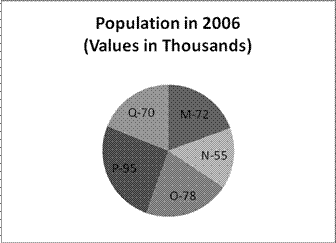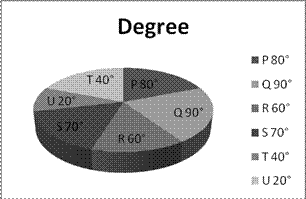# Mixed Data Interpretation

D.1-5)Cities 2005 2006 Literate% Illiterate% Literate% Illiterate% M 45 55 55 45 N 50 50 72 28 O 35 65 50 50 P 55 45 60 40 Q 50 50 60 40

Q.1) What is the total illiterate population rise or fall in 2006 compare to 2005?

a) 2500 increase

b) 2400 decrease

c) 1800 increase

d) 2100 decrease

e) No changes

 2005 Total Literate Illiterate % Population % Population M 64000 45 28800 55 35200 N 40000 50 20000 50 20000 O 60000 35 21000 65 39000 P 80000 55 44000 45 36000 Q 50000 50 25000 50 25000

 2006 Total Literate Illiterate % % M 72000 55 39600 45 32400 N 55000 72 39600 28 15400 O 78000 50 39000 50 39000 P 95000 60 57000 40 38000 Q 70000 60 42000 40 28000

Total illiterate in 2005 = 35200 + 20000 + 39000 + 36000 + 25000

= 155200.

Total illiterate in 2006 = 32400 + 15400 + 390000 + 38000 + 28000

= 152800

Illiterate population decrease in 2006 is = 155200 – 152800 = 2400.

Q.2) What is the total literate population in 2006 in all the cities together (in thousands)?

a) 207.1

b) 217.2

c) 209.62

d) 307.65

e) 198.42

b

Literate in 2006 =

 2006 Total Literate % M 72000 55 39600 N 55000 72 39600 O 78000 50 39000 P 95000 60 57000 Q 70000 60 42000

= 39600 + 39600 + 39000 + 57000 + 42000 = 217200.

Which is 217.2 thousands.

Q.3) What is the ratio between the literate population of city M in 2006 and the illiterate population of city P in 2005?

a) 10 : 11

b) 13 : 10

c) 9 : 7

d) 11 : 10

e) 6 : 7

d

M literate in 2006 =

 M 72000 55% 39600

P illiterate n 2005 =

 P 80000 45% 36000

Ratio = 39600 : 36000 = 11 : 10

Q.4) What is the average literate population in 2005?

a) 27760

b) 42730

c) 36480

d) 18860

e) 24000

a

Total Illiterate population in 2005 =

 2005 Total Literate Illiterate % Population % Population M 64000 45 28800 55 35200 N 40000 50 20000 50 20000 O 60000 35 21000 65 39000 P 80000 55 44000 45 36000 Q 50000 50 25000 50 25000

= 28800 + 20000 + 21000 + 44000 + 25000

= 138800

Average = 138800/5  = 27760

Q.5) What is the percentage rise in literate population of city Q from the year 2005 to 2006?

a) 65%

b) 55%

c) 68%

d) 54%

e) 73%

c

Literate in 2005 in city Q = 25000

In 2006 = 42000

Increase = 42000 – 25000 = 17000

% of increase = 17000/25000  × 100 = 68%

D.6-10) Study the following information carefully and answer the questions given below:

Six different companies P, Q, R, S, T and U make two items I and II. The total number of items produced by these six companies is 2,00,000. The cost of production of each item is Rs.20,000. The distribution of the total production by these companies is given in the following pie-chart and the table shows the ratio of production of Item I to that of Item II and the percentage profit earned by these companies on each of these items.Company Ratio of Production Percent Profit earned Item I Item II Item I Item II P 4 1 30 24 Q 3 2 15 25 R 2 3 10 12 S 1 2 25 30 T 1 3 15 20 U 2 3 20 15

Q.6) What is the profit earned by Company R on Item II?

a) 5.8 crore

b) 7.8 crore

c) 6.8 crore

d) 4.8 crore

e) None of these

d

According to question,

Profit earned by company R on Item II,

(60°)/(360°)  ×200000× 3/5×20000×12/100=4.8 crore

Ans=d) 4.8 crore

Q.7) What is the total cost of production of Item I by companies S and T together?

a) 17.04 crore

b) 27.04 crore

c) 37.04 crore

d) 47.04 crore

e) None of these

c

According to question,

Total cost of  production of item I by companies S and T together,

((70°)/(360°)×200000×1/3×20000)+((40°)/(360°)×200000×1/4×20000)=25.93 crore+11.11 crore

=37.04 crore

Ans=c) 37.04 crore

Q.8) What is the ratio of the cost of production of Item II by Company P to the cost of production of Item I by Company Q?

a) 8 : 27

b) 27 : 8

c) 15 : 3

d) 3 : 15

e) None of these

a

According to question,

P :Q=(1/5×(80°)/(360°))  :(3/5×(90°)/(360°))=8 :27

Ans=a) 8 :27

Q.9) What is the total of the profit earned by Company U on production of Item II and the profit of Company S on production of Item I?

a) 8.48 crore

b) 6.48 crore

c) 5.48 crore

d) 4.48 crore

e) None of these

a

According to question,

U+S=((20°)/(360°)×200000×3/5×20000×15/100)+((70°)/(360°)×200000×1/3×20000×25/100)

=2 crore+6.48 crore=8.48 crore

Ans=a) 8.48 crore

Q.10) What is the total of the profit earned by Company ‘T’on production of Item I and the profit of Company P on production of Item II?

a) 4.94 crore

b) 5.94 crore

c) 6.94 crore

d) 7.5 crore

e) None of these